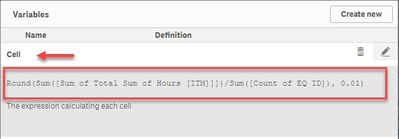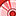# New to Qlik Sense

If you’re new to Qlik Sense, start with this Discussion Board and get up-to-speed quickly.

Announcements
Skip the ticket, Chat with Qlik Support instead for instant assistance.
cancel
Showing results for
Did you mean:Creator II

## Conditional colour formatting calculated measure/attribute

I would like to apply conditional colour formatting in my (pivot)table for a calculated measure/attribute.

I understood you can do this in "Background Color Expression" under the attribute measure? Though my condition is not straightforward as well as it is for a calculated attribute.  (see attachment for the explanation) How to set up the expression?  And how to create faded colors?

Labels (1)
• ### Qlik Sense

2 Solutions

Accepted SolutionsSpecialist II

It is working now, I will explain the steps done to reach the solution:

• New sheet added, its name: Pivot -> Table and Data Analysis
• Copied into the New Sheet the Pivot table (from FCO per market); then the Pivot table was converted into a table by dragging on top  Table control.
• By reading these topics the expression to calculate the cells figures are created.
• The expression to calculate the cells' value was worked out, it looks like this:

``Aggr((Sum([Sum of Total Sum of Hours [ITM]]])/Sum([Count of EQ ID])), Market, FCO)``

• As this expression is too long, and we need it for the background color expression the variable Cell was created• We worked out the expression calculating the SUM of all the Cells by Market and FCO, yes, it is also an Aggr() function, the expression looks like this:

``Aggr(Sum(Aggr((Sum([Sum of Total Sum of Hours [ITM]]])/Sum([Count of EQ ID])), Market, FCO)), FCO)``

• Actually, the Pivot table total the Avg(), so the formula become:

``Aggr(Avg(Aggr((Sum([Sum of Total Sum of Hours [ITM]]])/Sum([Count of EQ ID])), Market, FCO)), FCO)``

• As this expression is very long, and will become part of the expression resolving the Cell's color, we created the variable Total. The total expression evolved as explained next.
• If you look at the table, these columns Totals Sum and Avg are not repeating across the Market+FCO rows, this is resolved by another Aggr() function allowing us to repeat them (thanks Qlik); this topic really help Compute a subtotal value with aggr on all rows? ; the new expression is:

``````Aggr(nodistinct
Aggr(Avg(Aggr((Sum([Sum of Total Sum of Hours [ITM]]])/Sum([Count of EQ ID])), Market, FCO)), FCO)
, FCO
)``````

• We updated the expression of the variable Total with the latest finding!• If we look at the color expression, it has 3 main elements, the screenshot identifies them:• We already have the Cell and Total variables, next we created the Ratio variable!• Now we are able to update the color expression based on these variables; We tested it on the Ratio column of the test table.

``````=if(\$(Cell)>\$(Total),
ColorMix1(RangeMin(\$(Ratio) - 1,1), Argb(255,255,230,230), Argb(255,255,26,26)),
If(\$(Cell)<\$(Total),
ColorMix1(-(\$(Ratio) - 1), Argb(255,230,255,230), Argb(255,26,255,26)),
White()))``````

• By implementing variables (Cell, Total and Ratio) with complex expressions with hundreds of parenthesis opening and closing we simplified future expressions, like the background color!
• We assigned the new Background Color expression to the Pivot table, and it worked, with a GlitchThe Glitch is with the Totals row, it should have white background, I blame this to rounding, I did not try to figure out how to round it properly; the figures in the Totals row are generated by Qlik, our total figure was generated with Aggregation and unfortunately they did not match 😞

Attached is the new version of the QVF file.

Arnaldo Sandoval
A journey of a thousand miles begins with a single step.Specialist II

This is the application QVF file

Arnaldo Sandoval
A journey of a thousand miles begins with a single step.
16 RepliesSpecialist III

Use the Colormix1 function.  You will need to test for red, green, and blue scenarios.  For the red scenario, Colormix1(Value,White(),Red())  will produce shades of reds.Creator II
Author

Thanks, but what should be the expression? Since I need to compare it against the total average of the workorderEmployeeSpecialist III

If(Sum([Sum of Total Sum of Hours[ITM]]])/Sum([Count of EQ ID]) > Sum(Total [Sum of Total Sum of Hours[ITM]]])/Sum(Total [Count of EQ ID]),

Colormix1((Sum([Sum of Total Sum of Hours[ITM]]])/Sum([Count of EQ ID])/Max(Aggr(Sum([Sum of Total Sum of Hours[ITM]]])/Sum([Count of EQ ID]),[Sum of Total Sum of Hours[ITM]]]))),White(),Blue()),

If(Sum([Sum of Total Sum of Hours[ITM]]])/Sum([Count of EQ ID]) < Sum(Total [Sum of Total Sum of Hours[ITM]]])/Sum(Total [Count of EQ ID]),

Colormix1((Sum([Sum of Total Sum of Hours[ITM]]])/Sum([Count of EQ ID])/Max(Aggr(Sum([Sum of Total Sum of Hours[ITM]]])/Sum([Count of EQ ID]),[Sum of Total Sum of Hours[ITM]]]))),White(),Green()),

White()))Specialist II

Hi @Kirsten

I found your problem challenging, so I played with it, managing to color the table's cells based on the conditions you explained, I attached the solution QVF for you to review; a summary of my steps are:

• Created a mock-up of your table, obviously my cells are direct numbers.• On the Background color expression for each column/field I used an expression like the one below:
``````=if(W2>Round(Avg(TOTAL W2),0.01),
ColorMix1(RangeMin(W2/Round(Avg(total W2),0.01)-1,1), Argb(255,255,230,230), Argb(255,255,26,26)),
If(W2<Round(Avg(TOTAL W2), 0.01),
ColorMix1(-(W2/Round(Avg(total W2),0.01)-1), Argb(255,230,255,230), Argb(255,26,255,26)),
White()))``````
• I Round to round to two decimal places the Avg(), perhaps you should round your cells figures as well.
• I used the ColorMix1 function, it takes 3 parameters: value, first-color, last-color; if value is bigger than 1 (one) it did not returned any color, so I added RangeMin() function only when the Wx value is more than 100% bigger than its Avg(Wx).
• Just notice the differences with the value in the ColorMix1() function when Wx > Avg(Wx) and otherwise.
• Defining the color ranges with the ARGB() function was more rewarding rendering gradient colors.
• I used this Colors Picker page to pick up the ranges used, I converted the Hex codes to Decimal using this page Hex -> Dec

Attached is my sample project.

Hope this helps,

Arnaldo Sandoval
A journey of a thousand miles begins with a single step.Creator II
Author

Oh wow @ArnadoSandoval  thanks! It looks impressive. Thank you for that. I looked at the qvf and the code. My data structure is slightly different with a calculated measure in it. The calculated measure is the same as Avg in your code, this calculated measure is:  Sum([Sum of Total Sum of Hours [ITM]]])/Sum([Count of EQ ID]). When I add this calculated measure in the code it gives an error. FCO in my code is the same as W (W for workorder is more clear, I changed it to W to clarify) . QVF is added for clarification -> see attachment

=if(FCO>Round(Sum([Sum of Total Sum of Hours [ITM]]])/Sum([Count of EQ ID])(TOTAL FCO),0.01),

ColorMix1(RangeMin(FCO/Round(Sum([Sum of Total Sum of Hours [ITM]]])/Sum([Count of EQ ID])(total W2),0.01)-1,1), Argb(255,255,230,230), Argb(255,255,26,26)),

If(FCO<Round(Sum([Sum of Total Sum of Hours [ITM]]])/Sum([Count of EQ ID])(TOTAL FCO), 0.01),

ColorMix1(-(FCO/Round(Sum([Sum of Total Sum of Hours [ITM]]])/Sum([Count of EQ ID])(total W2),0.01)-1), Argb(255,230,255,230), Argb(255,26,255,26)),

White()))Creator II
Author

@ArnadoSandoval  what do I need to change in the code  in the message above to have it working? It is currently not fully working it returns an error when I add the calculated metric: Sum([Sum of Total Sum of Hours [ITM]]])/Sum([Count of EQ ID])Specialist II

I do not know yet, it is a pivot table, interesting challenge !!!

Regards,

Arnaldo Sandoval
A journey of a thousand miles begins with a single step.Specialist II

It is working now, I will explain the steps done to reach the solution:

• New sheet added, its name: Pivot -> Table and Data Analysis
• Copied into the New Sheet the Pivot table (from FCO per market); then the Pivot table was converted into a table by dragging on top  Table control.
• By reading these topics the expression to calculate the cells figures are created.
• The expression to calculate the cells' value was worked out, it looks like this:

``Aggr((Sum([Sum of Total Sum of Hours [ITM]]])/Sum([Count of EQ ID])), Market, FCO)``

• As this expression is too long, and we need it for the background color expression the variable Cell was created• We worked out the expression calculating the SUM of all the Cells by Market and FCO, yes, it is also an Aggr() function, the expression looks like this:

``Aggr(Sum(Aggr((Sum([Sum of Total Sum of Hours [ITM]]])/Sum([Count of EQ ID])), Market, FCO)), FCO)``

• Actually, the Pivot table total the Avg(), so the formula become:

``Aggr(Avg(Aggr((Sum([Sum of Total Sum of Hours [ITM]]])/Sum([Count of EQ ID])), Market, FCO)), FCO)``

• As this expression is very long, and will become part of the expression resolving the Cell's color, we created the variable Total. The total expression evolved as explained next.
• If you look at the table, these columns Totals Sum and Avg are not repeating across the Market+FCO rows, this is resolved by another Aggr() function allowing us to repeat them (thanks Qlik); this topic really help Compute a subtotal value with aggr on all rows? ; the new expression is:

``````Aggr(nodistinct
Aggr(Avg(Aggr((Sum([Sum of Total Sum of Hours [ITM]]])/Sum([Count of EQ ID])), Market, FCO)), FCO)
, FCO
)``````

• We updated the expression of the variable Total with the latest finding!• If we look at the color expression, it has 3 main elements, the screenshot identifies them:• We already have the Cell and Total variables, next we created the Ratio variable!• Now we are able to update the color expression based on these variables; We tested it on the Ratio column of the test table.

``````=if(\$(Cell)>\$(Total),
ColorMix1(RangeMin(\$(Ratio) - 1,1), Argb(255,255,230,230), Argb(255,255,26,26)),
If(\$(Cell)<\$(Total),
ColorMix1(-(\$(Ratio) - 1), Argb(255,230,255,230), Argb(255,26,255,26)),
White()))``````

• By implementing variables (Cell, Total and Ratio) with complex expressions with hundreds of parenthesis opening and closing we simplified future expressions, like the background color!
• We assigned the new Background Color expression to the Pivot table, and it worked, with a GlitchThe Glitch is with the Totals row, it should have white background, I blame this to rounding, I did not try to figure out how to round it properly; the figures in the Totals row are generated by Qlik, our total figure was generated with Aggregation and unfortunately they did not match 😞

Attached is the new version of the QVF file.

Arnaldo Sandoval
A journey of a thousand miles begins with a single step.Tags
Community Browser